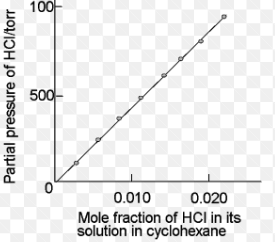# Solubility of gases in liquids

There’s dissolution of gases in liquids, but in general, to a little extent only. When the gases dissolve in liquid, gas molecules’ ability to freely move all over solvent’s volume is highly restricted.

So, if solvent’s volume is very small, as frequently the case is, efficiently, the gas is being compressed.

Due to these two effects, there is a decrease in the “entropy of the gas”, which is genrally not compensated by the decrease in entropy because of the mix of 2 types of molecules. Such procedures highly limit the solubility of gases in liquids.

Factors Which Affects “The Solubility of Gases in Liquids”:

Nature of solvent and gas:

• Easily those gases which are liquefiable are much more soluble as compared to dioxygen and dihydrogen.
• Gases having the capability of going through the chemical reaction along with H2O (water) have more solubility in water as compared to other solvents.

Temperature Effect:

• “The solubility of gases in liquids” is inversely proportional to temperature which means when solubility of gases in liquids goes down, there’s a temperature rise. When it’s dissolved, the molecules of the gas exist in liquid phase; moreover, dissolution process can be similarly considered with respect to condensation and the heat is ejected or evolved in this very process.
• The process of dissolution does include dynamic equilibrium as per our understanding and therefore, it must follow “Le Chatelier’s Principle.” Dissolution belongs to exothermic process, so the solubility must go down with temperature increase.

Effect of pressure:Fig 1

• When talking about solubility of gases in liquids – the temperature and pressure, both have a huge effect on it. With increase in pressure, the solubility of gas does increase.
• For gases solution present in solvent, observe the system as shown in image 1 in figure (a). In this, lower portion contains solution and the upper portion comprises of T and P. Presume this system that this is in dynamic equilibrium state that is, under such conditions, gaseous particles’ rate leaving and entering solution phase is equal.
• Now in figure (b), by increasing the P over solution phase by compressing gas in very small volume. This will result in increment in gaseous particles’ number per unit volume present over the solution plus also affect the rate at which gaseous particles are hitting solution’s surface to enter it. Unless a new equilibrium is achieved, there’ll be an increase in the solubility of gas. Also, the gas pressure will increase and therefore the solubility.

Henry’s Law:

A quantitative relation between solubility of a gas in the solvent and pressure was first given by Henry, which is called the Henry’s Law.

Henry’s Law is defined as when a constant temperature is in action, the (S) solubility of the gas in a liquid is ( α) directly proportional to (P) pressure of gas.

S α P

∴ S = KP

Where,

K = Henry’s constant

In Dalton theory, it was also concluded that the solubility of gases in liquids is independent and is a function of partial gas’s pressure.

Mostly used common form of Henry’s law defines that “partial pressure of the gas in (p) vapour phase is proportional to gas’ mole fraction (x) in a solution” and is represented as:

p = K in the suffix H * xFrom the graph, you can see different gases consist of different K in the suffix H values at same temperature. So, the conclusion says that higher value of K to the suffix H at some given P, lower is solubility of gases in liquid.